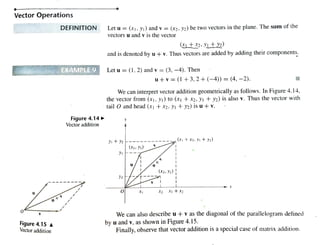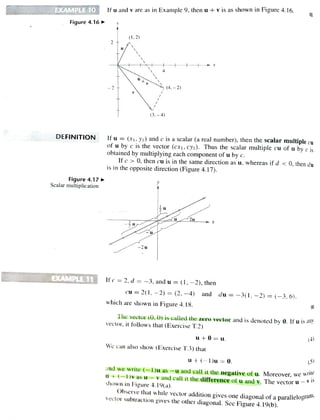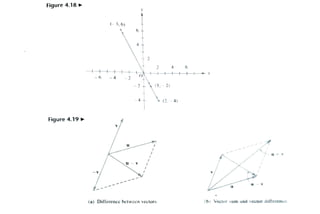Se está descargando tu SlideShare. ×

# 3 Vector Operations.pdf

Anuncio
Anuncio
Anuncio
Anuncio
Anuncio
Anuncio
Anuncio
Anuncio
Anuncio
Anuncio
AnuncioCargando en…3
×

1 de 3 Anuncio

# 3 Vector Operations.pdf

vector

vector

Anuncio
Anuncio

### 3 Vector Operations.pdf

1. 1. Vector Operations DEFINITION Let u = (1, y1) and v = (x2. y2) be two vectors in the plane. The sum of the vectors u and v is the vectoor and is denoted by u +v. Thus vectors are added by adding their components. EXAMPLE9 Let u = (1,2) and v = =(3, -4). Then u+V = (1+3, 2+ (-4)) = (4, -2). We can interpret vector addition geometrically as follows. In Figure 4.14, the vector from (x1, y1) to (X + X2, y + y2) is also v. Thus the vector with tail O and head (x1 +X2, yi + y2) is u+v. Figure4.14 Vector addition i+2 (XtX2y+y2) u +v (X2, y2) y2 O X2 1tX2 X u + V Figure 4.15 A Vector addition We can also describe u + v as the diagonal of the parallelogram detined by u and v, as shown in Figure 4.15. Finally, observe that vector addition is a special case of matrix addition.
2. 2. EXAMPLE10 Ifu and v are as in Example 9, then u +v is as shown in Figure 4.16. Figure4.16 (1.2) X + u ( 4 . - 2) (3,-4) of u by c is the vector (cx|, cy1). Thus the scalar multiple cu of u by c is obtained by multiplying each component of u by c. Ifc> 0, then cuis in the same direction as u, whereas if d < 0, then du is in the opposite direction (Figure 4.17). DEFINITION Ifu = (x1. y1) and c is a scalar (a real number), then the scalar multiple cu Figure4.17 Scalar multiplication 2u -2u EXAMPLE11 Ifc = = 2, d = =-3, and u = (1, -2), then cu = 2(1, -2) = (2, -4) and du = -3(1,-2) = (-3,6). which are shown in Figure 4. 18. The vector (0, 0) is called the zero vector and is denoted by 0. If u is any vector, it follows that (Exercise T.2) (4) u+0= u. We can also show (Exercise T.3) that (5) ut-Du=0. and we write (1)u as -u and eall it the negative of u. Moreover, we wr u+-1 v as uv and call it the differenceof u and v. The vectoru- shown in Figure 4.19(a). Observe that while vector addition gives one diagonal of a parallelogran vector suburaction gives the other diagonal. See Figure 4.19(6). V 1S
3. 3. Figure 4.18 (-3,6) 6 6 4 (1.-2) (2-4) Figure 4.19 u V (b) Vector sum and vector ditference. (a) Difference between vectors.